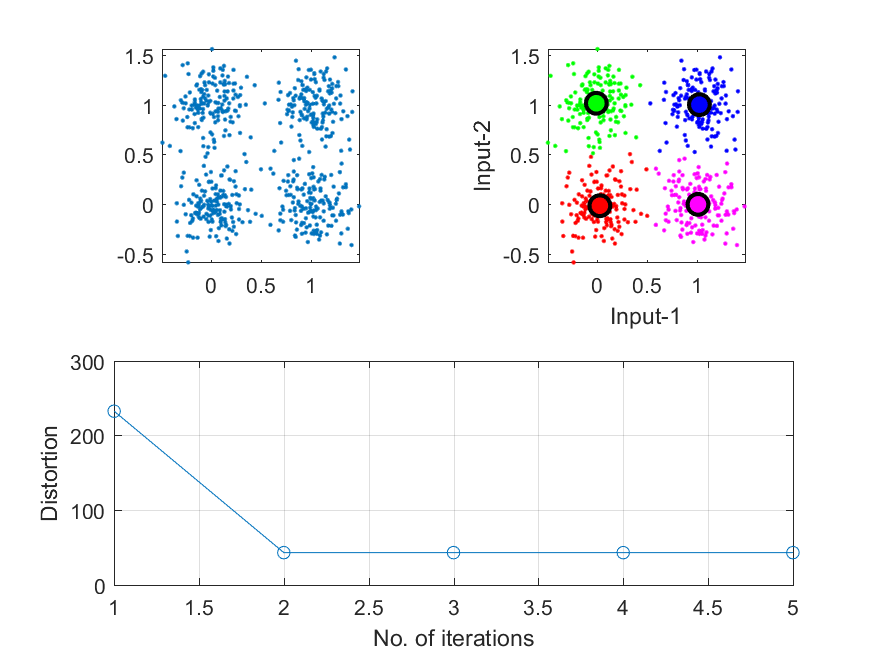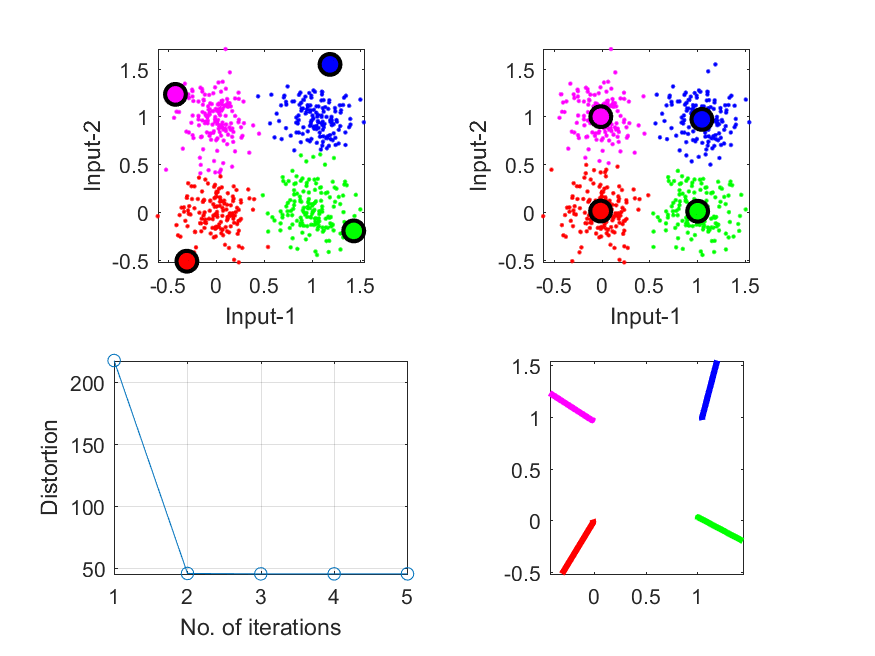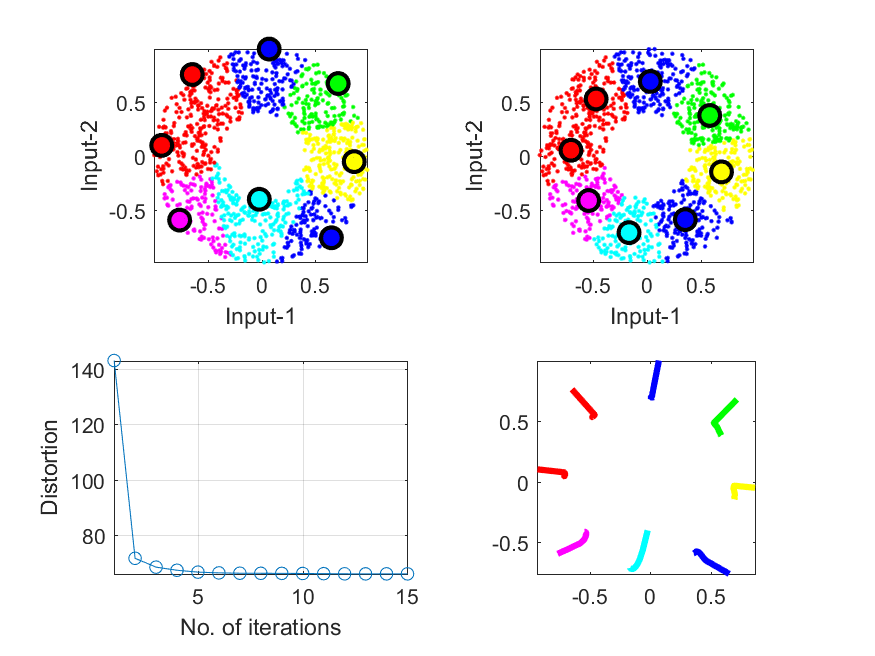## 3-3 K-means Clustering

[english][all]

(請注意：中文版本並未隨英文版本同步更新！)

• 資料壓縮：以少數的資料點來代表大量的資料，達到資料壓縮的功能。
• 資料分類：以少數代表點來代表特定類別的資料，可以降低資料量及計算量，並可以避免雜訊的不良影響。

E = Sp=1m ep

J(X; C, A) = Sxi in Gp|xi-cp|2,

1. 當C（群中心）固定時，我們可以很容易地找到對應的A（資料分群方式），使J(X; C, A)的值為最小。此最佳的A值，即代表最佳的分群方式，因此若將每個資料點歸類到距離最近的代表點，即可完成此目標。
2. 當A（資料分群方式）固定實，我們也可以很快地找到對應的C（群中心），使J(X; C, A)的值為最小。此最佳的C集合，代表每個代表點至其組員的距離平方和為最小，因此此最佳的C集合即是每一群的平均點。

1. 隨機選取 m 個資料點，將之分別視為 m 個群聚的群中心，這就是C。
2. 由固定的C，產生最佳的A。換句話說，對每一個資料點x，尋找與之最接近的群中心，並將x加入該群聚。
3. 計算目標函數 J(X; C, A)，如果保持不變，代表分群結果已經穩定不變，所以可以結束此疊代方法。
4. 再由固定的U，產生最佳的C。跳回第2個步驟。

Example 1: kMeans01.m% ====== Get the data set DS = dcData(5); subplot(2,2,1); plot(DS.input(1,:), DS.input(2,:), '.'); axis tight, axis image % ====== Run kmeans centerNum=4; [center, U, distortion, allCenters] = kMeansClustering(DS.input, centerNum); % ====== Plot the result subplot(2,2,2); vqDataPlot(DS.input, center); subplot(2,1,2); plot(distortion, 'o-'); xlabel('No. of iterations'); ylabel('Distortion'); grid onExample 2: kMeans02.m% ====== Get the data set DS = dcData(5); centerNum=4; % ====== Get initial cluster centers initCenter=vqCenterInit(DS.input, centerNum); subplot(2,2,1); vqDataPlot(DS.input, initCenter); % ====== Run k-means to get the final cluster centers [center, U, distortion, allCenters] = kMeansClustering(DS.input, initCenter); subplot(2,2,2); vqDataPlot(DS.input, center); % ====== Plot the distortion subplot(2,2,3); plot(1:length(distortion), distortion, 'o-'); xlabel('No. of iterations'); ylabel('Distortion'); grid on; axis tight % ====== Plot the moving paths of the centers subplot(2,2,4); for i=1:centerNum xData=allCenters(1,i,:); xData=xData(:); yData=allCenters(2,i,:); yData=yData(:); line(xData, yData, 'color', getColor(i), 'lineWidth', 3); end box on; axis image• 左上圖是啟始的群中心和分群的結果。
• 右上圖是最後的群中心和分群的結果。
• 左下圖是目標函數J(X; C, A)隨著疊代次數而遞減的情況。
• 右下圖是群中心雖著疊代次數而移動的路徑。

Example 3: kMeans03.m% ====== Get the data set DS = dcData(2); centerNum=8; % ====== Get initial cluster centers initCenter=vqCenterInit(DS.input, centerNum); clf; subplot(2,2,1); vqDataPlot(DS.input, initCenter); % ====== Run k-means to get the final cluster centers [center, U, distortion, allCenters] = kMeansClustering(DS.input, initCenter); subplot(2,2,2); vqDataPlot(DS.input, center); % ====== Plot the distortion subplot(2,2,3); plot(1:length(distortion), distortion, 'o-'); xlabel('No. of iterations'); ylabel('Distortion'); grid on; axis tight % ====== Plot the moving paths of the centers subplot(2,2,4); for i=1:centerNum xData=allCenters(1,i,:); xData=xData(:); yData=allCenters(2,i,:); yData=yData(:); line(xData, yData, 'color', getColor(i), 'lineWidth', 3); end box on; axis image• 這個方法只能保證J(X; C, A)越來越小，但無法保證能夠找到J(X; C, A)的絕對最小值，因此若時間許可，應該由不同的啟始群中心，多跑幾次，然後再選取最好的結果。
• 啟始群中心對於最後的分群結果有很大的影響，因此如何以很快的方法找到比較好的群中心，就變成重要的研究課題。以上述方法來說，若要選取 c 個起始中心典，常用的選取方法有下列幾種：
1. 從資料裡面隨意選出 m 點資料
2. 找出離所有資料平均值最遠的 m 個資料點
3. 找出離所有資料平均值最近的 m 個資料點
4. 找出距離平方和最小的 m 個資料點
但是這些找出起始群中心點的方法也都無法保證達到更好的目標函數值。如果計算時間不是太久，建議在選取啟始群中心時，多試用不同的方法，找出多組啟始群中心，同時進行 k-means，最後再以目標函數最小的結果來作為最後分群的結果。
• 另一個可能發生的問題，就是在進行 k-means 的過程中，有可能出現一個空的群聚，此群聚是空集合，沒有資料點。碰到這種情況，一個簡單的解決方式，就是直接將另一個群聚拆解為兩個，這樣才能保持群聚數目的不變。至於要找那一個群聚來切成兩個，可已有下列標準：
1. 找資料點最多的群聚
2. 找貢獻於目標函數最大的群聚
• 我們前述的方法是先找群中心，然後進行反覆疊代。我們也可以先分群，然後再反覆疊代，也可以得到類似的效果。

1. 隨機選取c的起始點，將之分別視為c個群聚的群中心
2. 對每一個資料點x，尋找與之最接近的群中心，並將x加入該群聚，隨即計算新的群聚中心（該群聚中原有的資料點加上x後的平均向量）
3. 檢查每一個資料點目前與之最接近的群聚中心是否和他的群聚分配一致，如果不是，則回到步驟二，反覆疊代，直到收斂。

• 適用於資料特性隨時間而變的情況。
• 計算簡單，適用於硬體實現。

Data Clustering and Pattern Recognition (資料分群與樣式辨認)